Select Page

# Trend Following & Factor Investing – Unexpected Cousins?

Questioning diversification benefits

April 2022. Reading Time: 10 Minutes. Author: Nicolas Rabener.

SUMMARY

• Trend following and beta-neutral factor investing are considered diversifying strategies
• However, since 2009 their correlations to stocks moved in tandem
• Both strategies had related performance drivers and risk exposures

INTRODUCTION

Asset classes seem easy to distinguish at first. For example, stocks and corporate bonds provide different exposure to the capital structure of companies. However, both suffer when the economy enters a recession as corporate earnings decrease and defaults increase. Perhaps not at the same time, but the exposure is more similar than most investors assume.

In other cases, the differences are less clear from the start. Take trend following and momentum investing, which seem to be pursuing the same strategy, ie following up and down trends of securities. Technically trend following is applied to a variety of asset classes via future contracts, while momentum means going long the best-performing and short the worst-performing stocks, although the latter can also be applied to other asset classes. The theme is the same, just the implementation is different.

In a previous research note (Building a Diversified Portfolio for the Long-Term), we came across data that indicated there might be a relationship between trend following and factor investing, which are usually regarded as uncorrelated strategies. If indeed they were correlated, then this would give rise to concerns as diversification benefits were less than commonly assumed.

In this research note, we will analyze the relationship between trend following and factor investing.

CORRELATIONS TO THE S&P 500

We focus on trend following in the classic sense, which implies going long and short a variety of asset classes like oil, gold, sugar, and so on. It is a systematic approach to exploiting trends and results in a diversified portfolio of asset classes. We use the SG Trend Index as a proxy, which is comprised of ten strategies that are equally weighted and rebalanced annually.

For factor investing we create a multi-factor portfolio comprised of the value, size, momentum, quality, and low volatility factors, which are equally weighted. The factors are created beta-neutral and based on the entire universe of stocks in the US with a market capitalization larger than \$1 billion.

As a first step in the analysis, we calculate the 12-month rolling calculation of trend following and the multi-factor portfolio to the S&P 500. The average correlation was approximately zero between 2002 and 2022, which indicates attractive diversifications benefits for an equity portfolio. However, the correlations oscillated strongly and occasionally were moderately positive to equities.

More interesting is that from 2006 onwards the correlations of trend following and the multi-factor portfolio to equities moved in tandem, which suggests that these were correlated themselves (read Smart Beta & Factor Correlations to the S&P 500).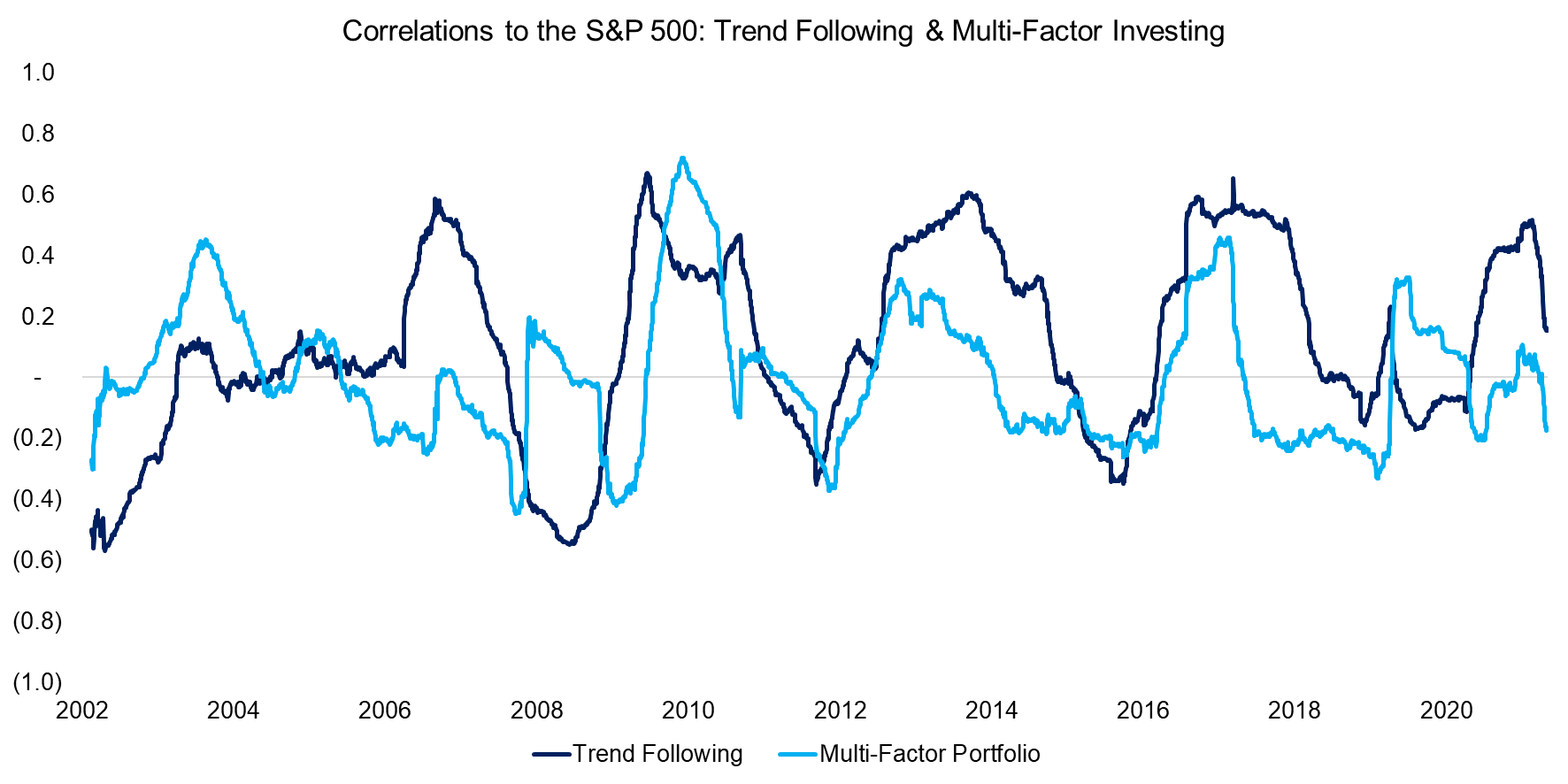Source: FactorResearch

CORRELATIONS OF EQUITY FACTORS TO TREND FOLLOWING

Next, we analyze the correlation of the beta-neutral equity factors to trend following. Given that the correlations of the five factors oscillated around 0, it is somewhat difficult to form a view by eyeballing the time series, except that there is not a single factor that had a strongly positive or negative correlation to trend following.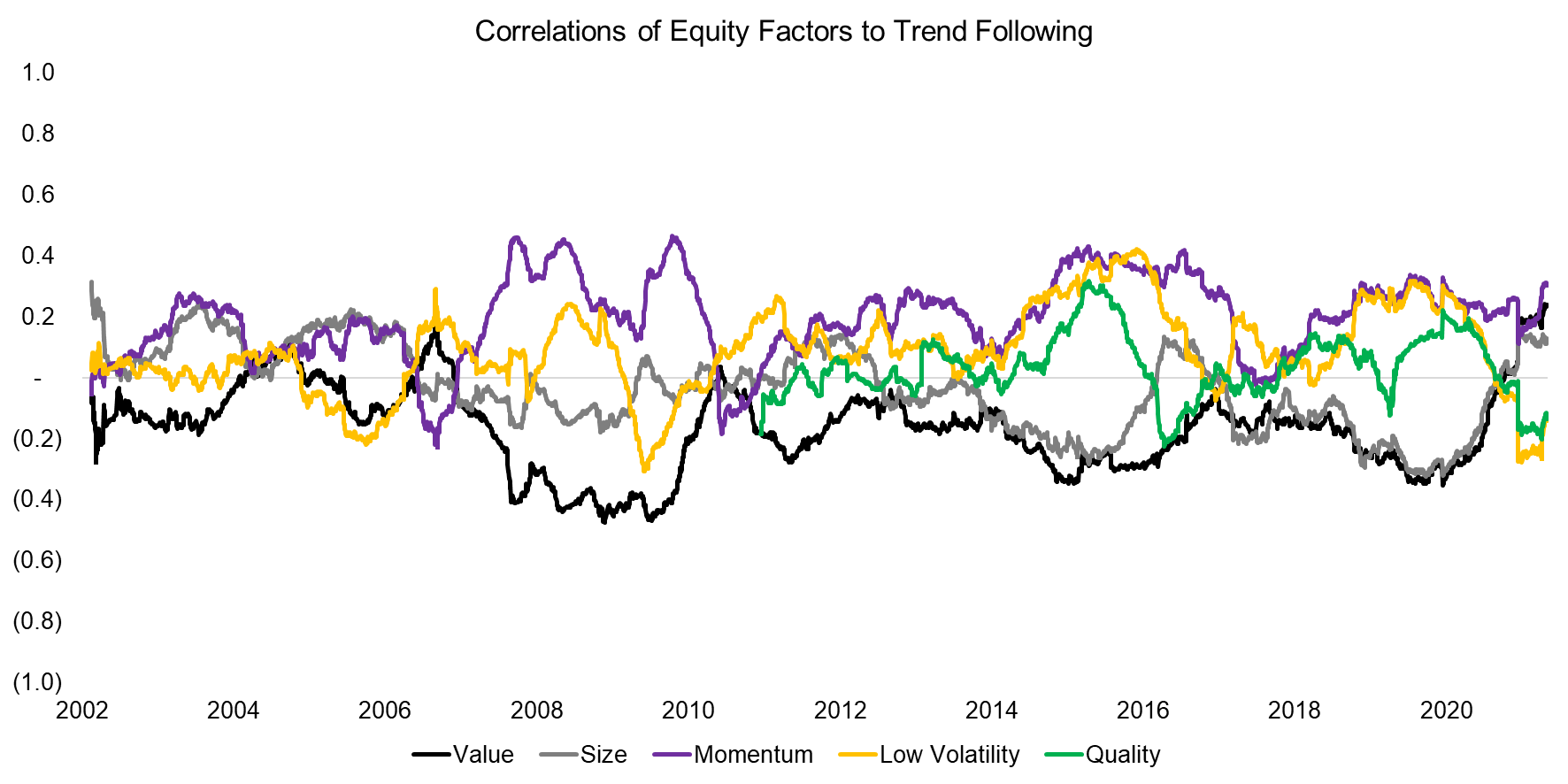Source: FactorResearch

Calculating the average correlations in the period from 2002 to 2022 confirms that these were close to zero, which indicates that the returns of factor investing and trend following are uncorrelated. Both strategies should be attractive from a diversification perspective for an equity portfolio.

However, we also observe that value and size were slightly negatively and momentum, low volatility, and quality factors slightly positively correlated. These two groups are distinct and usually negatively correlated with each other, eg when cheap small caps outperform, then the best-performing, high quality, and low volatility stocks tend to underperform.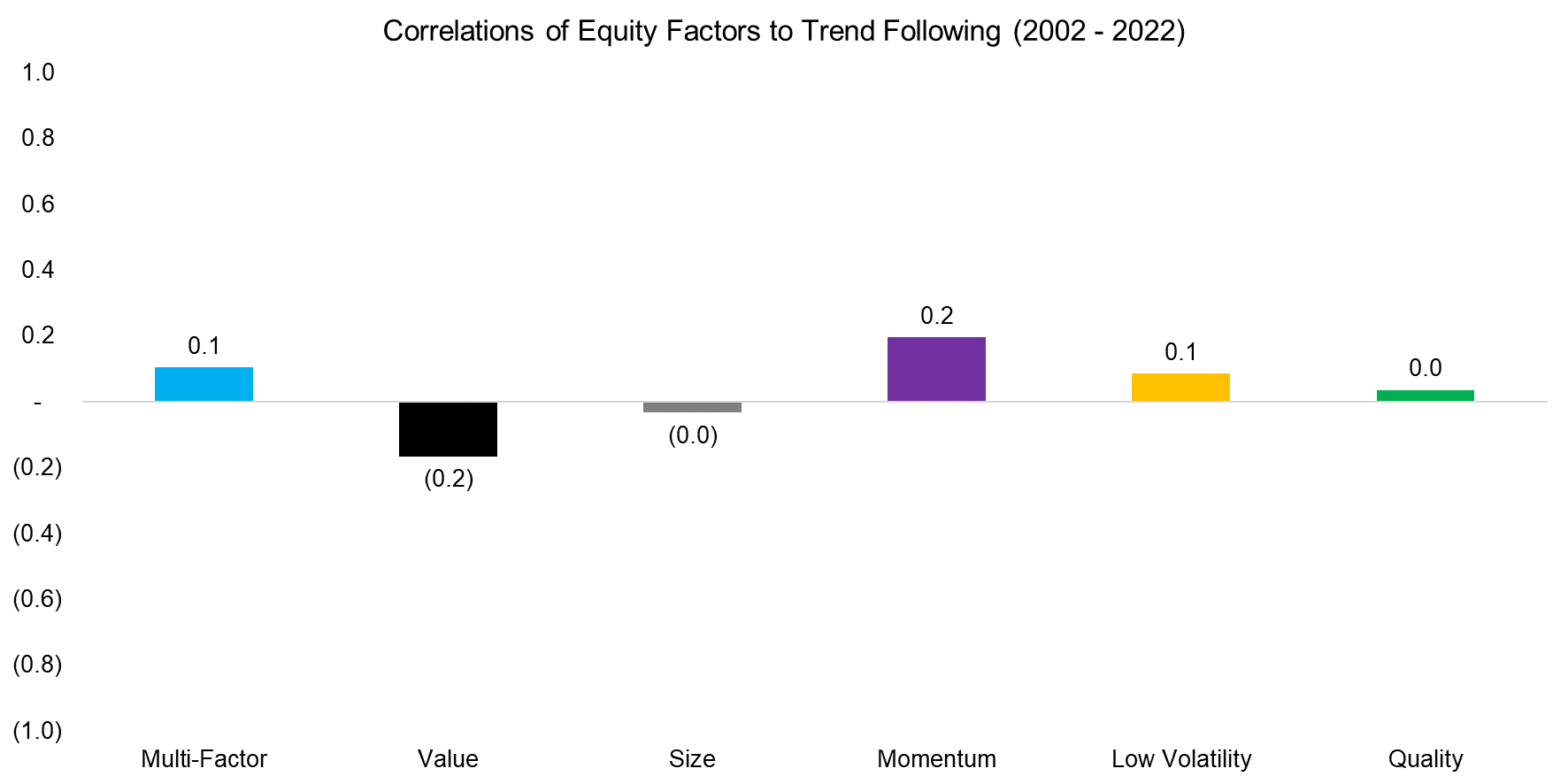Source: FactorResearch

TREND FOLLOWING VS MOMENTUM, QUALITY, AND LOW VOLATILITY FACTORS

We can create a multi-factor portfolio comprised of the momentum, quality, and low volatility factors and see if that explains the relationship with the trend following strategy seen earlier. And indeed, if we calculate the correlations of that multi-factor portfolio and trend following to the S&P 500, then the correlations moved even more in tandem, although only strongly since the global financial crisis in 2009 (read Multi-Factor Models 101).

Intuitively, this should be explained by the momentum factor and trend following strategy taking similar exposures as both are exploiting trends in the stock market and economy, eg momentum a long position in outperforming gold miners and trend following a long position in gold.

However, the quality and low volatility factors also exhibit similar trends in correlation to equities. The relationship between these two factors and trend following can be attributed to long positions in technology (quality factor) and utilities & real estate stocks (low volatility factor) over the last decade, which benefitted from decreasing interest rates. Trend following strategies were long bonds throughout most of the same period.

It seems that trend following and factor investing strategies have indirectly been providing the same risk exposure. Given that this is done via different instruments and portfolio construction methodologies, a simple correlation analysis fails to highlight these relationships.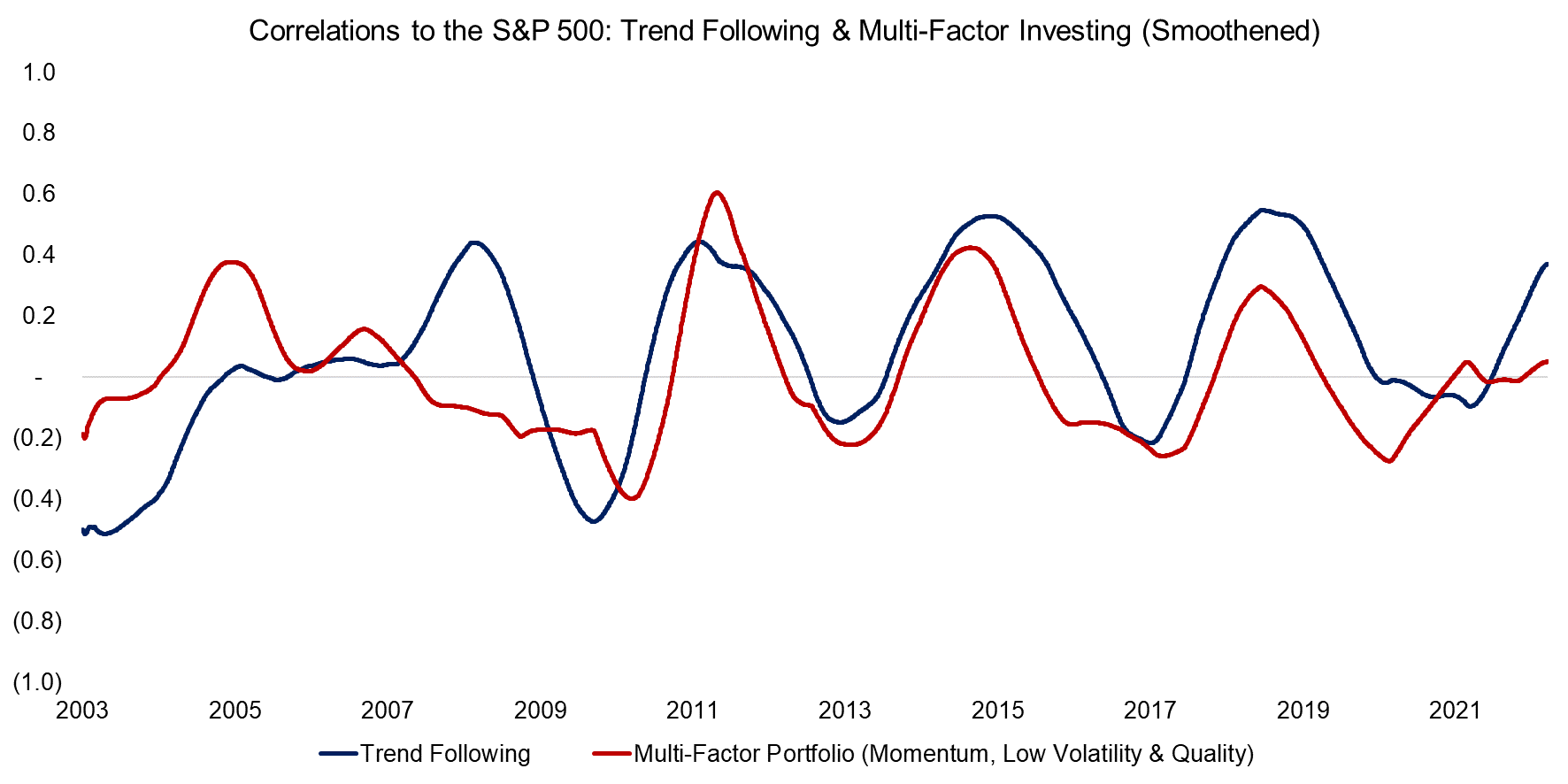Source: FactorResearch

PERFORMANCE OF TREND FOLLOWING & MULTI-FACTOR INVESTING

It is worth highlighting that the picture that emerges from the rolling correlation charts is not one that is expected by viewing the performance of trend following and factor investing.

The performance of trend following between 2002 and 2022 looks completely different from that of factor investing, even compared to a multi-factor portfolio comprised of momentum, low volatility, and quality factors. Partially this is due to the significantly higher volatility of trend following (try Finominal’s Portfolio Diagnostics for portfolio analysis).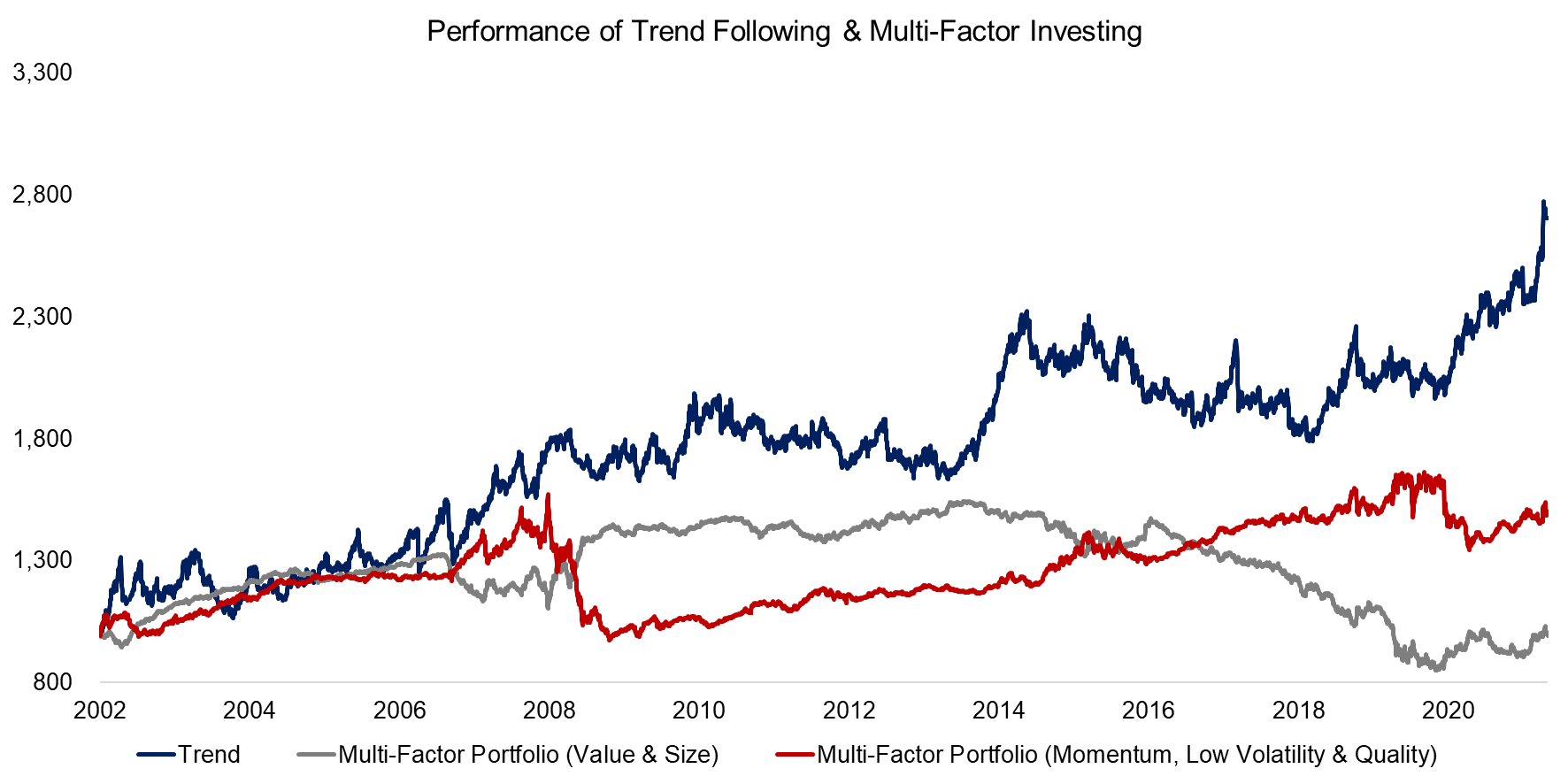Source: FactorResearch

FURTHER THOUGHTS

Although the correlation between trend following and factor investing was low on average, investors should be concerned that the correlations to equities frequently moved in tandem. Both strategies are used for generating diversification benefits for equity investors, but they had the same performance drivers for almost a decade.

Stated differently, an investor might question if he needs both strategies for a diversified portfolio, or is just doubling up on the same risk exposure.

RELATED RESEARCH

Building a Diversified Portfolio for the Long-Term

Managed Futures: Fast & Furious vs Slow & Steady

Liquid Alt Juggernauts: Worth their Salt?

60/40 Portfolios Without Bonds

Aging & Equities: Selling Stocks for the Long-Term

Creating Anti-Fragile Portfolios

Long Volatility Strategies: Hedge Funds vs DIY